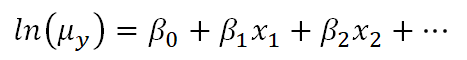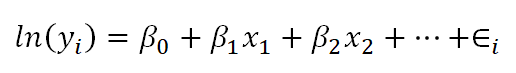# OptinMon 31 - GLMM

### R-Squared for Mixed Effects Models

August 21st, 2019 by

When learning about linear models —that is, regression, ANOVA, and similar techniques—we are taught to calculate an R2. The R2 has the following useful properties:

• The range is limited to [0,1], so we can easily judge how relatively large it is.
• It is standardized, meaning its value does not depend on the scale of the variables involved in the analysis.
• The interpretation is pretty clear: It is the proportion of variability in the outcome that can be explained by the independent variables in the model.

The calculation of the R2 is also intuitive, once you understand the concepts of variance and prediction. (more…)

### Regression Diagnostics in Generalized Linear Mixed Models

March 25th, 2019 by

What are the best methods for checking a generalized linear mixed model (GLMM) for proper fit?

This question comes up frequently.

Unfortunately, it isn’t as straightforward as it is for a general linear model.

In linear models the requirements are easy to outline: linear in the parameters, normally distributed and independent residuals, and homogeneity of variance (that is, similar variance at all values of all predictors).

### The Difference Between Link Functions and Data Transformations

September 24th, 2018 by

Generalized linear models—and generalized linear mixed models—are called generalized linear because they connect a model’s outcome to its predictors in a linear way. The function used to make this connection is called a link function. Link functions sounds like an exotic term, but they’re actually much simpler than they sound.

For example, Poisson regression (commonly used for outcomes that are counts) makes use of a natural log link function as follows:Clearly, there is not a direct linear relationship of the x variables to the average count, but there is a “sort of linear” relationship happening: a function of the mean of y is related to a linear combination of x variables. In other words, the linear model has now been generalized to a bigger type of situation.

This can lead to confusion, though, because on the surface it looks very similar to what happens when we transform the dependent variable in a linear model, like a linear regression.

The key thing to understand is that the natural log link function is a function of the mean of y, not the y values themselves.

### Transformations of Y

Below is a linear model equation where the original dependent variable, y, has been natural log transformed. That is, the natural log has been taken of each individual value of y, and that is being used as the dependent variable.The linear model with the log transformation is providing an equation for an individual value of ln(y). We could also write it as follows, where we are modeling the mean of ln(y) (note the error term is no longer present):This makes the difference a bit clearer. When we transform the data in a linear model, we are no longer claiming that y is normally distributed around a mean, given the x values — we are claiming that our new outcome variable, ln(yi), is normally distributed.

In fact, we often make this transformation specifically because the values of y do not appear to be normally distributed around their average.

In the case of the Poisson model, however, the link function does not change the distribution of the actual observations in some way to make them something other than Poisson distributed. Instead, the link function defines the relationship of the x variables directly to the mean of the Poisson distributed y. The individual observations then vary around this expected value accordingly.

### The mean of the log is not the log of the mean

As you may know, if you have used this kind of data transformation in a linear model before, you cannot simply take the exponent of the mean of ln(y) to get the mean of y.

You might be surprised to know, though, that you can do this with a link function. If you have specific values of your x variables, you can calculate the predicted average count, μy based on those x values by inversing the natural log:This ability to back-transform means (and regression coefficients) to a more intuitive scale is part of what makes generalized linear models so useful.

### Understanding Random Effects in Mixed Models

September 17th, 2018 by

In fixed-effects models (e.g., regression, ANOVA, generalized linear models), there is only one source of random variability. This source of variance is the random sample we take to measure our variables.

It may be patients in a health facility, for whom we take various measures of their medical history to estimate their probability of recovery. Or random variability may come from individual students in a school system, and we use demographic information to predict their grade point averages.

### What is the Purpose of a Generalized Linear Mixed Model?

September 10th, 2018 by

If you are new to using generalized linear mixed effects models, or if you have heard of them but never used them, you might be wondering about the purpose of a GLMM.

Mixed effects models are useful when we have data with more than one source of random variability. For example, an outcome may be measured more than once on the same person (repeated measures taken over time).

When we do that we have to account for both within-person and across-person variability. A single measure of residual variance can’t account for both.

### Models for Repeated Measures Continuous, Categorical, and Count Data

April 6th, 2015 by

Lately, I’ve gotten a lot of questions about learning how to run models for repeated measures data that isn’t continuous.

Mostly categorical. But once in a while discrete counts.

A typical study is in linguistics or psychology where (more…)